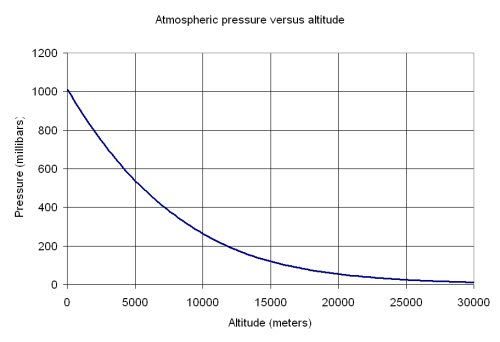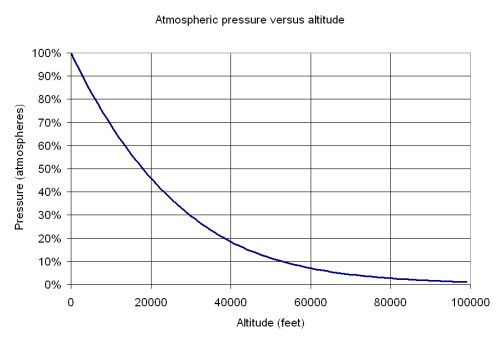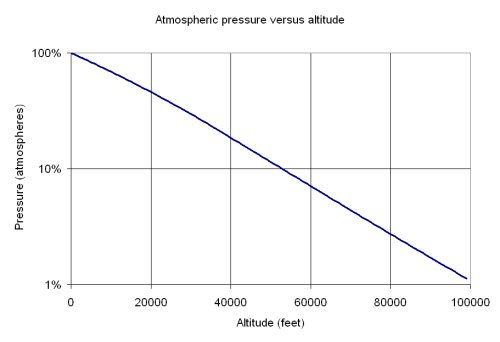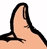# Atmospheric Pressure

This page is part of a larger discussion of power handling capability. Atmospheric pressure is important because lower air pressure means that voltage breakdown occurs at lower electric field strength.

There are many units of pressure, including millimeters of mercury (same as a Torr), inches mercury, inches of water, Torr, Pascals (and kilo-Pascals and mega-Pascals because a Pascal is so tiny). Let's review some of the conversions:

1 atmosphere=760 Torr 760 Torr

1 atmosphere=760 Torr (mm Hg) or 29.92 inches Hg) Thanks to Ken for the correction!

1 atmosphere=14.69 pounds per square inch (PSI)

1 atmosphere =101325 Pascals=101.325 kilo-Pascals (kPA)

1 atmosphere= 1.01325 bar=1013.25 millibar (mbar)

Now you can do your own conversions if you want to convert mbar to Torr for example. Altitude can be measured in feet, miles, meters, kilometers. One foot is 0.3048 meters.

The equations for pressure versus altitude are complicated, and the best resource for this is NASA, and we don't have them handy so they won't be presented here. Here we have downloaded data from the atmos.com web site in millimeters of mercury, then we converted the data to atmosphere and millibars.

Now we will show two plots of atmospheric pressure versus altitude. The first one is for fans of the metric system, in mbars versus meters:The second plot is for fans of American units, in atmospheres versus feet:Here's a "bonus" plot, where we've graphed the pressure on a log scale. You can see that it is almost a straight line, which implies that it is a negative exponential function. In reality it is a piecewise fifth-order polynomial (maybe we'll put the equations up some day).Now it's time for a Microwaves101 rule of thumb:At 100,000 feet, pressure is about 1% of what it is at sea level. For all intents and purposes, if your system has to operate at 100,000 feet, you can consider that it must perform in a vacuum (zero air pressure).

Author : Unknown Editor